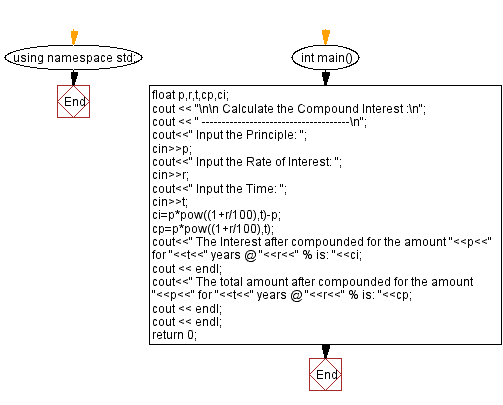﻿ C++ Exercises: Calculate Compound Interest - w3resource

# C++ Exercises: Calculate Compound Interest

## C++ Basic: Exercise-55 with Solution

Write a C++ program to enter P, T, R and calculate compound interest.

Sample Solution:

C++ Code :

``````#include<iostream>
#include<math.h>

using namespace std;

int main()
{
float p,r,t,cp,ci;
cout << "\n\n Calculate the Compound Interest :\n";
cout << " -------------------------------------\n";
cout<<" Input the Principle: ";
cin>>p;
cout<<" Input the Rate of Interest: ";
cin>>r;
cout<<" Input the Time: ";
cin>>t;
ci=p*pow((1+r/100),t)-p;
cp=p*pow((1+r/100),t);
cout<<" The Interest after compounded for the amount "<<p<<" for "<<t<<" years @ "<<r<<" % is: "<<ci;
cout << endl;
cout<<" The total amount after compounded for the amount "<<p<<" for "<<t<<" years @ "<<r<<" % is: "<<cp;
cout << endl;
cout << endl;
return 0;
}
``````

Sample Output:

```Calculate the Compound Interest :
-------------------------------------
Input the Principle: 20000
Input the Rate of Interest: 10
Input the Time: 1.5
The Interest after compounded for the amount 20000 for 1.5 years @ 10
% is: 3073.8
The total amount after compounded for the amount 20000 for 1.5 years @
10 % is: 23073.8
```

Flowchart:C++ Code Editor:

What is the difficulty level of this exercise?

﻿

## C++ Programming: Tips of the Day

Why is there no std::stou?

The most pat answer would be that the C library has no corresponding "strtou", and the C++11 string functions are all just thinly veiled wrappers around the C library functions: The std::sto* functions mirror strto*, and the std::to_string functions use sprintf.

Ref: https://bit.ly/3wtz2qA

We are closing our Disqus commenting system for some maintenanace issues. You may write to us at reach[at]yahoo[dot]com or visit us at Facebook